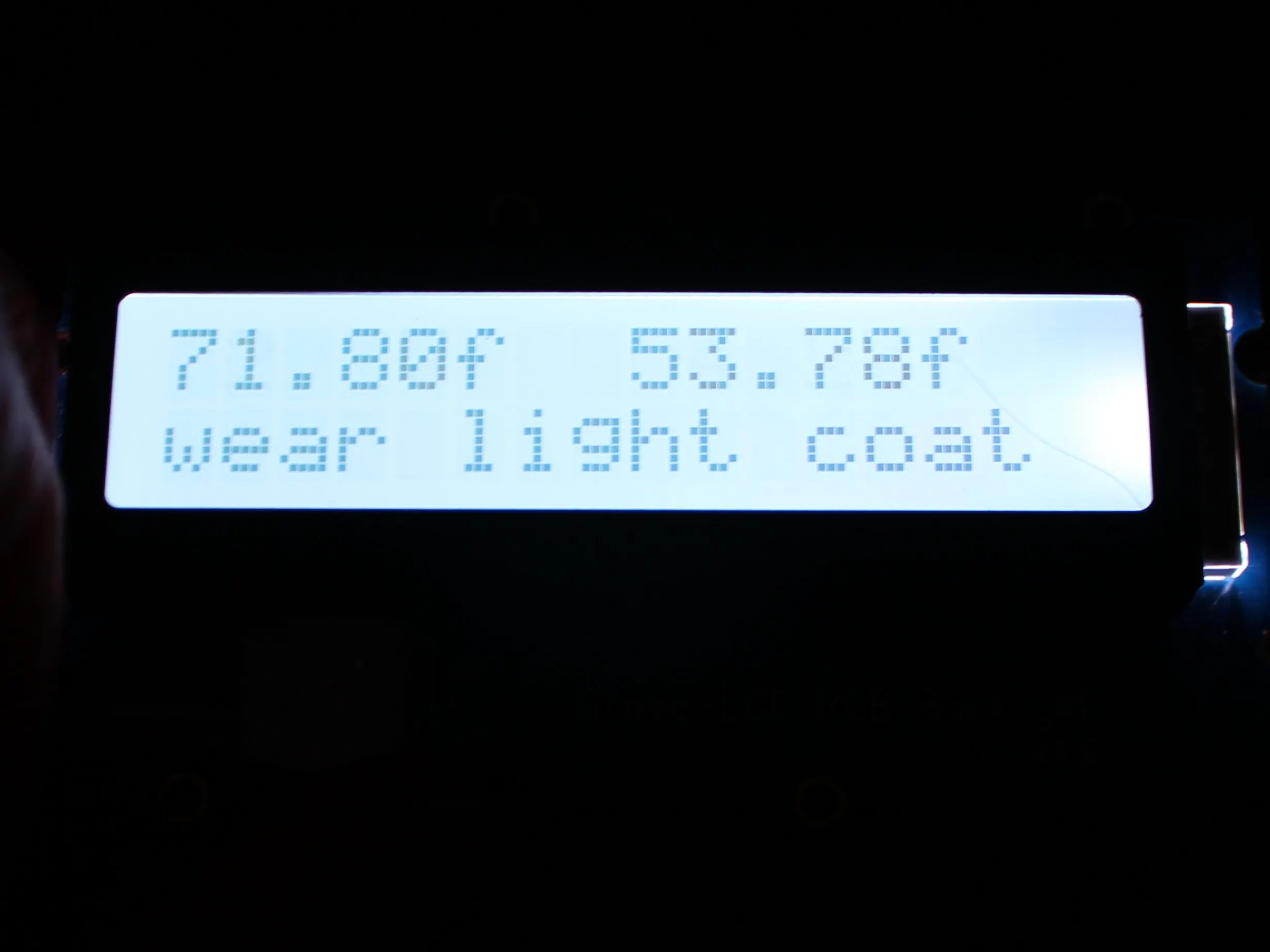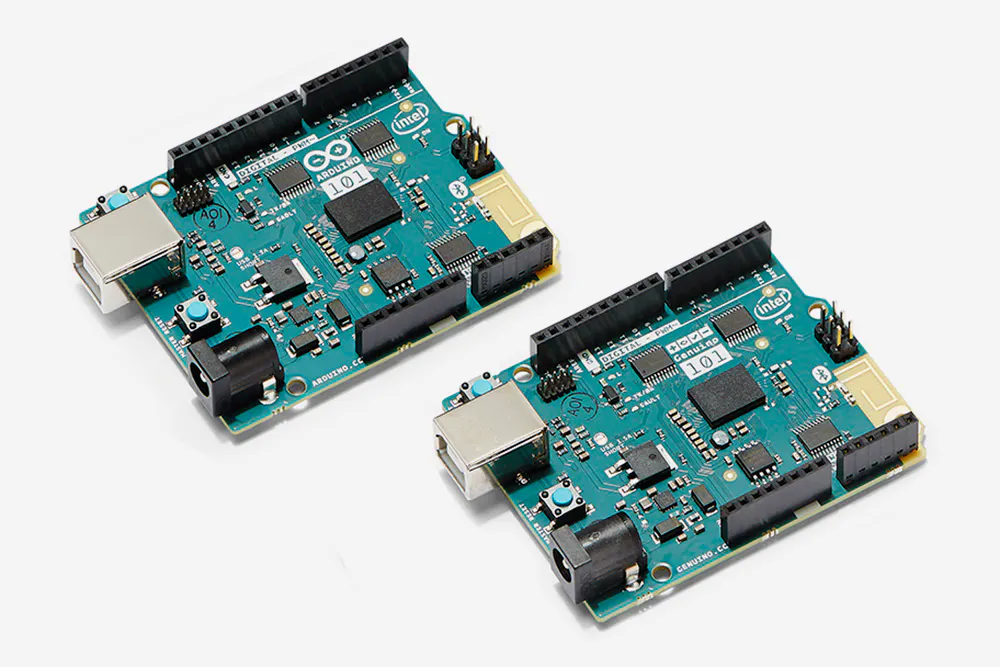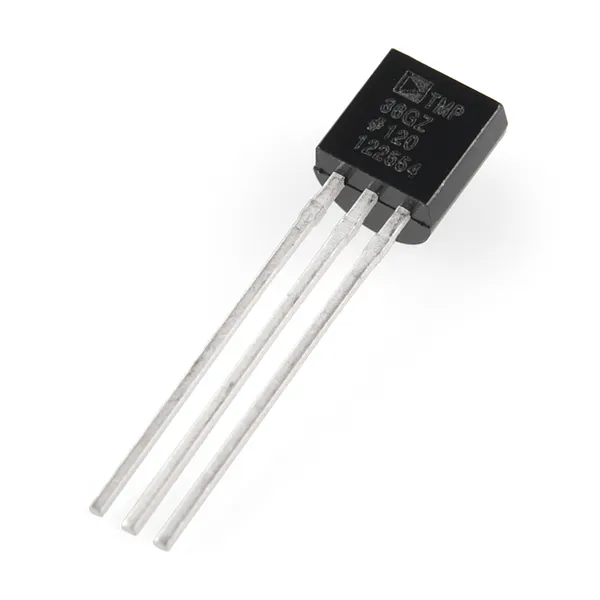Project tutorialArduino 101 Clothes Recommender © LGPL

Arduino 101 that tells you the inside and outside temperature and recommends what clothes to wear.

• 2,113 views
• 3 respects

Components and suppliesArduino 101 & Genuino 101
×1Temperature Sensor
×1
 Seeed Grove - RGB Backlight LCD - 16x2
×1
 Seeed Grove - Temperature Sensor
×1
 Seeed Grove - Button
×1
 Seeed Grove - Touch Sensor
×1

Apps and online services

Setup:

First you must install the library for the LCD screen as explained in my

In order to use the Grove LCD you'll need to download a library here, and move it to your Arduino>Libraries folder:

And for hardware connections, plug the LCD screen into any of the I2C ports, the button into D2, and the touch sensor into D3, and the temperature sensor into A1:

You must also connect pin 1 of the TMP 36 to 5v, pin 2 to A0, and pin 3 to GND. The pins are as follows:

Then just stick the TMP 36 out the window, and mount the rest however you want.

Code:

I won't explain the code in full because the code is heavily commented, but I will talk about 2 functions.

In the void loop, this while loop sets the background to the correct color, then sets the background color, decreases the value of the count var, delays 20 milliseconds, and reduces the count var by 1. Then it repeats, this will happen 50x since the count var = 50.

while (count > 0) {
if (background == 1) { //if the background int is 1:
R = 255; //set the variables for the background
G = 0;
B = 0;
}
else if (background == 2) { //else if the background int is 2:
R = 0; //set the variables for the background
G = 255;
B = 0;
}
else if (background == 3) { //else if the background int is 3:
R = 0; //set the variables for the background
G = 0;
B = 255;
}
else if (background == 4) { //else if the background int is 5:
R = 255; //set the variables for the background
G = 255;
B = 255;
}
lcd.setRGB(R, G, B);//set the background color of the lcd screen
count--;
delay(20);//delay 20 milliseconds
}

Moving on to the functions, the functions for finding the temperature are directly from the data sheets, and the others are as follows:

The checkButtons function creates a variable called b that is assigned the value of the button. Then if b is 1, which means the button is pressed, it will change to the next background unless it's on the last one (4) in which case it will start over at 1.

int checkButtons(int b1, int bc) {
int b = digitalRead(b1);//make the variable b what is read on b1
if (b == 1) { //if b (the reading of b1) is 1 (pressed)
if (background < 4) {
background++;
}
else if (background == 4) {
background = 1;
}
}
if (b == 1) {
if (cf == 0) {
cf = 1;
}
else if (cf == 1) {
cf = 0;
}
}
return background;// return the background int
return cf;//return the celsius/fahrenheit int
}

Controls:

To change between C and F press the touch sensor, and to change the background color press the button.

Product:

Once you have downloaded the code your project should look something like this:

Code

Clothes recomenderArduino
#include <Wire.h>    //include 2 libraries
#include "rgb_lcd.h"//for rgb lcd

#include <math.h>//include a library for math that will be done with the thermistor.

rgb_lcd lcd;

const int b1 = 2; //this is the button for the background
const int bc = 3; //this is the touch button that switches between fahrenheit and celsius

const int ot = A0;//this is the pin for the outside temp

float R;  //set the variables
float G; //for the color
float B;//of the background

int background = 4;//different values will make the rgb lcd a different color

int a;//original thermosistor value
int base = 3985;//base value of the thermistor
float c;//float for celsius
float f;//float for fahrenheit
float oc;//float for outside celsius
float of;//float for outside fahrenheit

int cf = 0;//0 for celsius, 1 for fahrenheit

float resistance;//float for the resistance of the thermistor

int i;//var for calling on function to find inside temp
int o;//var for calling on function to find outside temp

int cb;//int for calling on function to check the buttons

int count = 50;//int for looping the part that checks the color 50x

char* rec[]={"wear warm jacket","wear light coat","wear sweatshirt","wear long-sleves","wear a T-shirt"};//string for the cloathing recomendation
int re;//a var that will be used to determine which rec string to use

void setup() {
lcd.begin(16, 2);// set up the LCD's number of columns and rows:

pinMode(b1, INPUT); //define the buttons as input
pinMode(bc, INPUT);
}

void loop() {
cb = checkButtons(b1, bc);

while (count > 0) {
if (background == 1) { //if the background int is 1:
R = 255; //set the variables for the background
G = 0;
B = 0;
}
else if (background == 2) { //else if the background int is 2:
R = 0; //set the variables for the background
G = 255;
B = 0;
}
else if (background == 3) { //else if the background int is 3:
R = 0; //set the variables for the background
G = 0;
B = 255;
}
else if (background == 4) { //else if the background int is 5:
R = 255; //set the variables for the background
G = 255;
B = 255;
}
lcd.setRGB(R, G, B);//set the background color of the lcd screen
count--;
delay(20);//delay 5 milliseconds
}

int w =wetherRec(oc);

if (cf == 0) {
i = findC();
o = findOC();
lcd.clear();
lcd.setCursor(0, 0);
lcd.print(c);
lcd.print("c");
lcd.setCursor(8, 0);
lcd.print(oc);
lcd.print("c");
lcd.setCursor(0, 1);
if (re=1){
lcd.print(rec);
}
else if (re=2){
lcd.print(rec);
}
else if (re=3){
lcd.print(rec);
}
else if (re=4){
lcd.print(rec);
}
else if (re=5){
lcd.print(rec);
}
}

if (cf == 1) {
i = findF();
o = findOF();
lcd.clear();
lcd.setCursor(0, 0);
lcd.print(f);
lcd.print("f");
lcd.setCursor(8, 0);
lcd.print(of);
lcd.print("f");
lcd.setCursor(0, 1);
if (re=1){
lcd.print(rec);
}
else if (re=2){
lcd.print(rec);
}
else if (re=3){
lcd.print(rec);
}
else if (re=4){
lcd.print(rec);
}
else if (re=5){
lcd.print(rec);
}
}
count=50;
}

int findF() { // function to find fahrenheit
a = analogRead(A1); //find the original reading of the thermistor
resistance = (float)(1023 - a) * 10000 / a; //get the resistance of the sensor;
c = 1 / (log(resistance / 10000) / base + 1 / 298.15) - 273.15; //convert to Celsius
f = (c * (9 / 5) + 32); //convert to fahrenheit
return c;
return f;//return the fahrenheit
}

int findC() { // function to find celsius
a = analogRead(A1); //find the original reading of the thermistor
resistance = (float)(1023 - a) * 10000 / a; //get the resistance of the sensor;
c = 1 / (log(resistance / 10000) / base + 1 / 298.15) - 273.15; //convert to Celsius
return c;//return the celsius
}

int findOF() { //function to find the outside fahrenheit
int t = analogRead(ot);//create a variable to store the value of the outside tmp36 module
float voltage = (t / 1024.0) * 5.0;//convert the ADC reading to voltage
// the sensor changes 10 mV per degree celsius
// the datasheet says there's a 500 mV offset
oc = (voltage - .5) * 100;//convert to celsius
of = (oc * (9 / 5) + 32); //convert to fahrenheit
return oc;
return of;//return the outside fahrenhiet
}

int findOC() { //function to find the outside fahrenheit
int t = analogRead(ot);//create a variable to store the value of the outside tmp36 module
float voltage = (t / 1024.0) * 5.0;//convert the ADC reading to voltage
// the sensor changes 10 mV per degree celsius
// the datasheet says there's a 500 mV offset
oc = (voltage - .5) * 100; //convert to celsius
return oc;//return the outside celsius
}

int wetherRec(int oc){
if(oc<-5){//if the outside temp (in c) is less than -5
re=1;//change re to "wear warm jacket"
}
else if(oc<12){//etc...
re=2;
}
else if(oc<16){
re=3;
}
else if(oc<21){
re=4;
}
else{
re=5;
}
}

int checkButtons(int b1, int bc) {
int b = digitalRead(b1);//make the variable b what is read on b1
if (b == 1) { //if b (the reading of b1) is HIGH (pressed)
if (background < 4) {
background++;
}
else if (background == 4) {
background = 1;
}
}

if (b == 1) {
if (cf == 0) {
cf = 1;
}
else if (cf == 1) {
cf = 0;
}
}
return background;// return the background int
return cf;//return the celsius/fahrenheit int
}

SchematicsMarch 27, 2017

Members who respect this project

See similar projects
you might like

SMS alerts for arduino 101 BLE

Project tutorial by Alexis Santiago Allende

• 1,905 views
• 7 respects

(Updated) Digital Clock w/ Mirrored Display for UNO-R3 & 101

Project tutorial by LAGSILVA

• 993 views
• 3 respects

Smart Garbage Monitoring System Using Arduino 101

Project tutorial by Technovation

• 21,398 views
• 32 respects

Arduino 101 BLE App

Project in progress by Alexis Santiago Allende

• 10,796 views
• 51 respects

Arduino/Genuino 101 BLE Thermometer With TMP102 and Blynk

Project tutorial by Konstantin Dimitrov

• 7,877 views
• 1 comment
• 26 respects

Oled Display with Arduino 101

Project tutorial by Alexis Santiago Allende

• 6,084 views
• 1 comment
• 25 respects